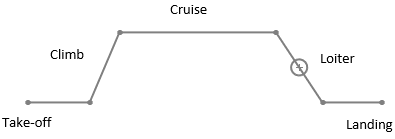Q.1
##### What does the following diagram represents?• a) Simple cruise mission profile
• b) Accelerated cruise
• c) Bomber mission
• d) Penetration strike
Q.2
##### For given mission leg ‘n’, mission leg weight fraction is _____
• a) Wn / Wn-1
• b) Wn / Wn-2
• c) Wn-1 / Wn+1
• d) Wn / Wn+1
Q.3
##### Which of the following is part of the fuel-fraction method?
• a) Developing lofting
• b) Empty weight fraction
• c) Calculating individual mission segment weight fraction
• d) Finding volume ratio
Q.4
• a) 150lb
• b) 150kg
• c) 60kg
• d) 60lb
Q.5
##### Choose appropriate option for cruise mission leg weight fraction.
• a) Breguet endurance formula
• b) Breguet range formula
• c) Cruise invert method
• d) Breguet approximation
Q.6
##### What will be the total fuel weight fraction for x-number of mission phases?
• a) Wf / W0 = 1 – (Wx / W0)
• b) Wf / W0 = 1 + (Wx / W0)
• c) Wf / W0 = 1 / (Wx / W0)
• d) Wf / W0 = 1 + (Wx / W0)
Q.7
##### What will be the weight fraction of the aircraft at final mission segment w.r.t. W0?
• a) Multiplication of individual weight fraction
• b) Division of individual weight fraction
• c) Addition of individual weight fraction
• d) Subtraction of individual weight fraction
Q.8
##### How will you determine climb mission weight fraction?
• a) Climb Endurance
• b) Loiter
• c) Always constant
• d) Cruise Speed
Q.9
##### If all the other factors remain unchanged and only aerodynamic efficiency of the aircraft is doubled then, what will be the cruise segment fuel weight fraction?
• a) Will increase
• b) Will decrease
• c) Will remain same
• d) Will be independent of aerodynamic efficiency
Q.10
• a) True
• b) False
Q.11
• a) 0.9216
• b) 0.9261
• c) 0.9289
• d) 0.9365
Q.12
##### An Aircraft has following mission profiles with their respective mission weight fraction. There isallowance of reserved fuel. Now, due to some reason loiter weight fraction has changed to 0.Now, with same amount of fuel as initial, will aircraft be able to perform loiter?
• a) Yes, loiter can be performed
• b) No, loiter cannot be performed
• c) Will not depend on change
• d) 12% less fuel will require